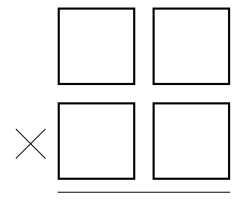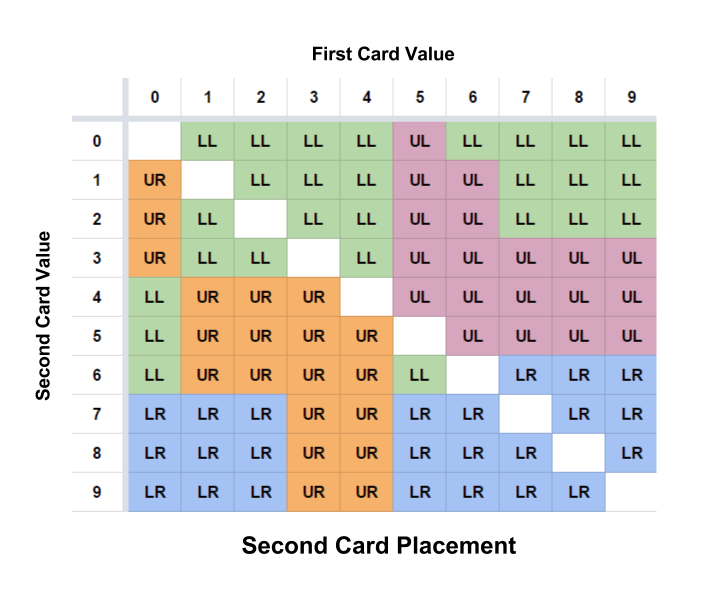Consider the following game. In front of you is a stack of 10 cards printed with the numbers 0 through 9, one per card. The stack is shuffled and, sight unseen, you draw a number from the top. You look at the number and place it somewhere in the multiplication equation below. You then draw another number, look at it, and place it somewhere else in the equation. You do that two more times, until all four slots are filled. Once a digit is placed, it can’t be moved, and it can’t be drawn again because it’s no longer in the stack.Your goal is to build a multiplication equation with the lowest possible product. What is the optimal strategy? And how much of this game is luck and how much is skill? In other words, how much does the expected product under the optimal strategy differ from simply placing the cards randomly?

## Solution:

First we’ll consider how to proceed with only pencil and paper, and then we’ll bring out the computer to determine how our strategy would play out for all possible sequences of four cards.

Here is the expectation for a randomly chosen $AB \times CD$ (relying on the linearity of expectation):

$E = E(100A\cdot C + 10A \cdot D + 10 B \cdot C + B \cdot D)$ $= 121 E(A\cdot C)$ $= 121 \times \frac{1}{90}\left(\sum_{m=0}^9 \sum_{n=0}^9 mn - \sum_{n=0}^9 n^2\right) = 121 \times \frac{58}{3} = 2339\frac{1}{3}$

Where should we put the first card—on the right or on the left (we’ll assume it goes in the top row, though of course it doesn’t matter)? Here’s a (non-airtight) argument that cards showing $4$ and below should go on the left, and the others on the right.

The expectation for starting with the number $m$, placing it top-left, and then placing the others randomly:

$E(m\mbox{ top-left}) = E(100m \cdot C + 10m \cdot D + 10B \cdot C + B \cdot D)$ $= 110 E(m \cdot C) + 11E(B \cdot C)$ $E(m \cdot C) = \left(\sum_{n=0}^9 \frac{1}{9} mn\right) - \frac{1}{9}m^2 = \frac{45m-m^2}{9}$ $E(B \cdot C) = \frac{1}{72}\left(\left(\sum_{n=0}^9\sum_{k=0}^9 nk\right) - \left(\sum_{n=0}^9 n^2\right) - \left( 2\times \sum_{n=0}^9 mn\right) + 2m^2 \right)$ $= \frac{1740-90m+2m^2}{72}$ $E(m\mbox{ top-left}) = 110 \times \frac{45m-m^2}{9} + 11 \times \frac{1740-90m+2m^2}{72}$ $= \frac{1595}{6} + \frac{2145}{4}m - \frac{143}{12}m^2$

Similarly, the expectation for placing $m$ top-right and then proceeding randomly:

$E = E(100A \cdot C + 10A \cdot D + 10C \cdot m + D \cdot m)$ $= 110\times \frac{1740-90m+2m^2}{72} + 11 \times \frac{45m-m^2}{9}$ $= \frac{7975}{3} - \frac{165}{2}m + \frac{11}{6}m^2$

Comparing these values allows us to see that placing a first number less than or equal to $4$ on the top-left is the best bet, while placing one greater than or equal to $5$ on the right is the best bet. This gives us a good, though admittedly not decisive, reason to think that whatever the optimal strategy is, it involves the same decision for the first move. That is important, because it greatly simplifies the problem.

There are $5040$ possible sequences of four cards, and $24$ possible ways to place the cards in any given sequence, and so it might seem that the space of possibilities you have to consider is unsurveyably vast. However, when you have placed the first card and have received your second, there are three places to choose from, and only $56$ sequences of third and fourth cards to consider. So you compute an expectation for each place, by assuming you’ve placed the second card there, and for each of the eight possible third cards, noting the lower of the expectations of placing it in the two remaining open places (computed by averaging the products produced by placing each of the seven remaining cards in the last open place). Average those lower expectations to get the expectation of placing the second card in that place (and then playing optimally). Choose the place with the least expectation, and you will already have done the figuring needed to place the third card when you receive it.

You’re done!

The code below quickly finds the optimal strategy for every possible four-card sequence. It confirms our educated guess that we should put first-cards showing $4$ and under on the left and $5$ and above on the right, and it yields an overall expectation of about $1056.84$, which is about $45.18\%$ of the expectation of playing randomly.

Here’s a chart detailing the crux of the strategy, which is the placement of the second card (in the chart, “UL” means upper-left, etc.).As you can see, the strategy resists easy summary—there’s no simple intuition behind it. However, a few observations can make sense of it.

In general we want low numbers to end up on the left and high ones on the right. An interesting exception is a first-card value of $0$, where the upper-right card will have an out-sized effect on the product. So we profit by taking the opportunity to put a second low card there right away. With a second card of $4$, $5$, or $6$, we should hold out for a smaller number in the crucial upper right (of which there’s at least a $9/14$ chance in the final two cards), while not risking having to put an even higher number in the lower left (of which there’d be at least a $9/14$ chance).

And we profit from matching very low numbers with not-so-high numbers, and not-so-low numbers with very high ones. For example, $19\times 35$ is $665$, whereas $15\times 39$ is $585$. This explains why when the first card is $1$ a second-card $9$ goes in the lower right, whereas when the first card is $3$ a second-card $5$ goes in the upper right.

And if you get a $5$ (which goes upper-right) followed by a $6$, you have a $9/14$ chance of getting at least one of $7$, $8$, and $9$ in the remaining two cards; so it pays to take the hit and place the $6$ on the left, treating it as a very high “low card,” and leaving open the lower right for the expected higher card. Similar reasoning explains why it pays to treat $4$ as a not-so-high high second card with a low first card (apart from $0$), and to treat $5$ as a not-so-low low second card given a high first card.

### Code:

# fivethirtyeight.com Riddler https://fivethirtyeight.com/features/can-you-outsmart-our-elementary-school-math-problems/

# A situation is a tuple (A,B,C,D), representing AB*CD, where each element
# is a digit or -1 to represent an unfilled position.

# Strategy is a "dictionary" that contains optimal moves for pairs of
# a situation and a number (the number on the new card):
# E.g., Strategy[((2,-1,-1,9),4)] = 2 means that 4 should go in the third
# (python tuple index 2) spot.
Strategy = {}

# Expectation is a dictionary from situations to the expectation of them
# given optimal strategy.
Expectation = {}

def Process(situation):
# Determine the optimal strategies for playing with this situation
# and any next card, and generate the expectation of playing
# optimally starting from this situation.

global Strategy, Expectation
accum =  0
num_newcards = 0
for newcard in range(10):
if newcard in situation:
continue
least_expected_product = [-1,10000]
for i in range(4):
if situation[i] == -1:
sitlist = list(situation)
sitlist[i] = newcard
newsit = tuple(sitlist)
if not -1 in newsit:
Expectation[newsit] = \
(10*newsit+newsit)*(10*newsit+newsit)
elif not newsit in Expectation:
Process(newsit)
E = Expectation[newsit]
if E < least_expected_product:
least_expected_product = [i,E]
Strategy[(situation,newcard)] = least_expected_product
accum += least_expected_product
num_newcards += 1
Expectation[situation] = accum/num_newcards

Process((-1,-1,-1,-1))

print("Optimal strategy expects",Expectation[(-1,-1,-1,-1)])